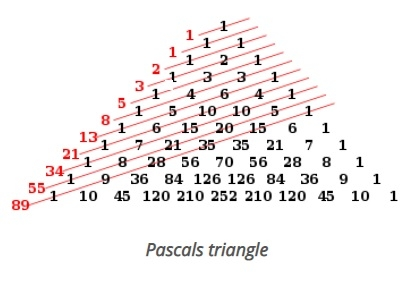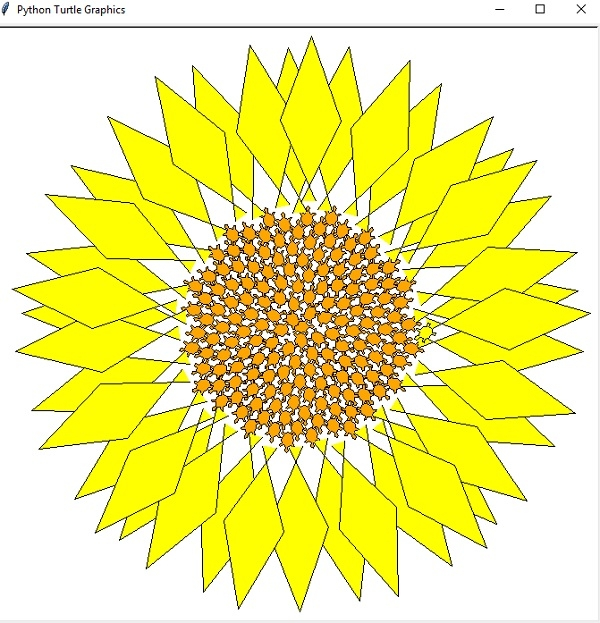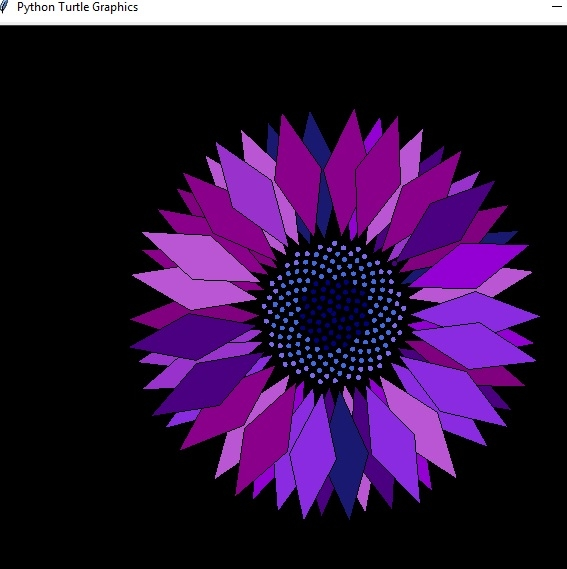# Phyllotaxis pattern in Python?

## What is phyllotaxis pattern?

When we go back, in our botany classes and the plant world, phyllotaxis means the arrangement of flowers, leaves or seeds on a plant stem, similar to the one found in Fibonacci spiral. Based on Fibonacci sequence, the Fibonacci spiral is a set of numbers that follows a pattern similar on pascal’s triangle. The Fibonacci sequence numbers are something like - 1, 1, 2, 3, 5, 8, 13, 21, 34, 55, 89, 144, etc. So the Fibonacci sequence number is the sum of its previous numbers.

## Fibonacci spirals

We generally look for symmetry and patterns to understand the objects around us. Without realizing it our eyes are seeing the Fibonacci sequences, or in the case of a sunflower head, a Fibonacci spiral.

## SolutionSunflower spiral

## Example Code

import math
import turtle

def PhyllotacticPattern( t, petalstart, angle = 137.508, size = 2, cspread = 4 ):
"""print a pattern of circles using spiral phyllotactic data"""
# initialize position
turtle.pen(outline=1,pencolor="black",fillcolor="orange")
# turtle.color("orange")
phi = angle * ( math.pi / 180.0 )
xcenter = 0.0
ycenter = 0.0

# for loops iterate in this case from the first value until < 4, so
for n in range (0,t):
theta = n * phi

x = r * math.cos(theta) + xcenter
y = r * math.sin(theta) + ycenter

# move the turtle to that position and draw
turtle.up()
turtle.setpos(x,y)
turtle.down()
# orient the turtle correctly
if n > petalstart-1:
#turtle.color("yellow")
drawPetal(x,y)
else: turtle.stamp()

def drawPetal( x, y ):
turtle.up()
turtle.setpos(x,y)
turtle.down()
turtle.begin_fill()
#turtle.fill(True)
turtle.pen(outline=1,pencolor="black",fillcolor="yellow")
turtle.right(25)
turtle.forward(100)
turtle.left(45)
turtle.forward(100)
turtle.left(140)
turtle.forward(100)
turtle.left(45)
turtle.forward(100)
turtle.up()
turtle.end_fill() # this is needed to complete the last petal

turtle.shape("turtle")
turtle.speed(0) # make the turtle go as fast as possible
PhyllotacticPattern( 200, 160, 137.508, 4, 10 )
turtle.exitonclick() # lets you x out of the window when outside of idle

## SolutionWith a small change in your above program, you result could be displaced something like (give custom color and alter some values):